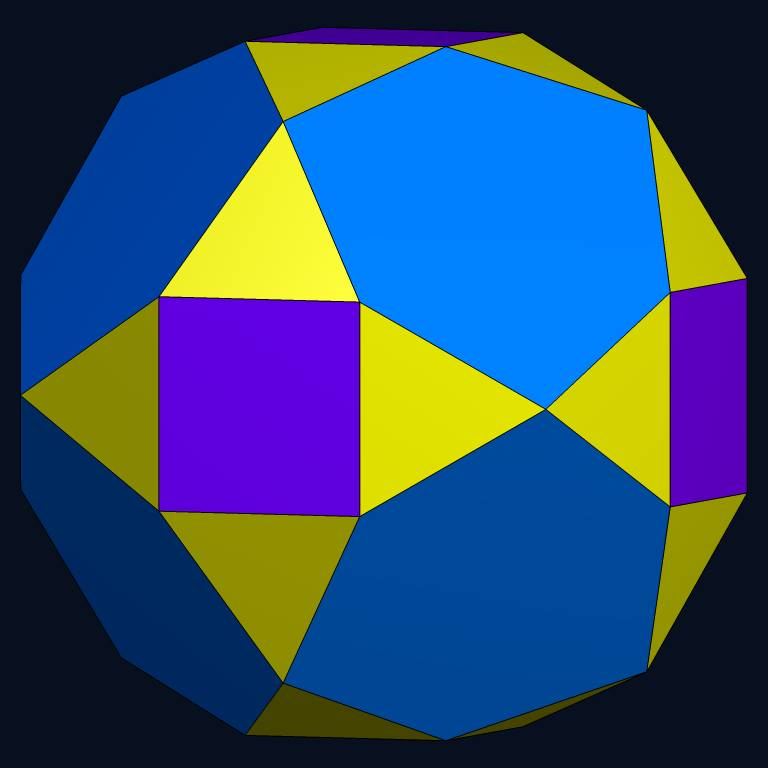Acronym ... Name dodeca-augmented rhombicuboctahedron© Vertex figure [3,4,3,H], [3,h,3,h] Coordinates (V/2, 1/2, 1/2)         & all permutations, all changes of sign (vertex inscribed a3o4x, a = sqrt(5/2)) (F/2, F/2, 0)           & all permutations, all changes of sign (vertex inscribed o3b4o, b = (3+sqrt(5))/sqrt(8)) where F = f+x = ff, V = 2f Confer 30-augmented srid   expanded 12-augmented girco

The non-regular hexagons {(h,H)3} are shield hexagons. Their vertex angles are alternatingly h = arccos[-1/4] = 104.477512° resp. H = arccos[-(3 sqrt(5)-1)/8] = 135.522488°.

Incidence matrix according to Dynkin symbol

ao3ob4xo&#zx   → height = 0
a = HH = sqrt(5/2) = 1.581139 (pseudo edge)
b = hh = (3+sqrt(5))/sqrt(8) = 1.851230 (pseudo edge)
x = hH = 1

o.3o.4o.     | 24  * |  2  2 | 1 1  2  [3,4,3,H]
.o3.o4.o     |  * 12 |  0  4 | 0 2  2  [3,h,3,h]
-------------+-------+-------+-------
.. .. x.     |  2  0 | 24  * | 1 0  1
oo3oo4oo&#x  |  1  1 |  * 48 | 0 1  1
-------------+-------+-------+-------
.. o.4x.     |  4  0 |  4  0 | 6 *  *
ao3ob ..&#xz |  3  3 |  0  6 | * 8  *  {(h,H)3}
.. .. xo&#x  |  2  1 |  1  2 | * * 24